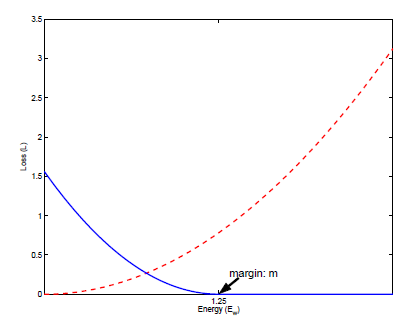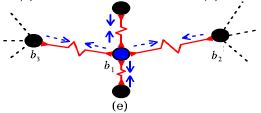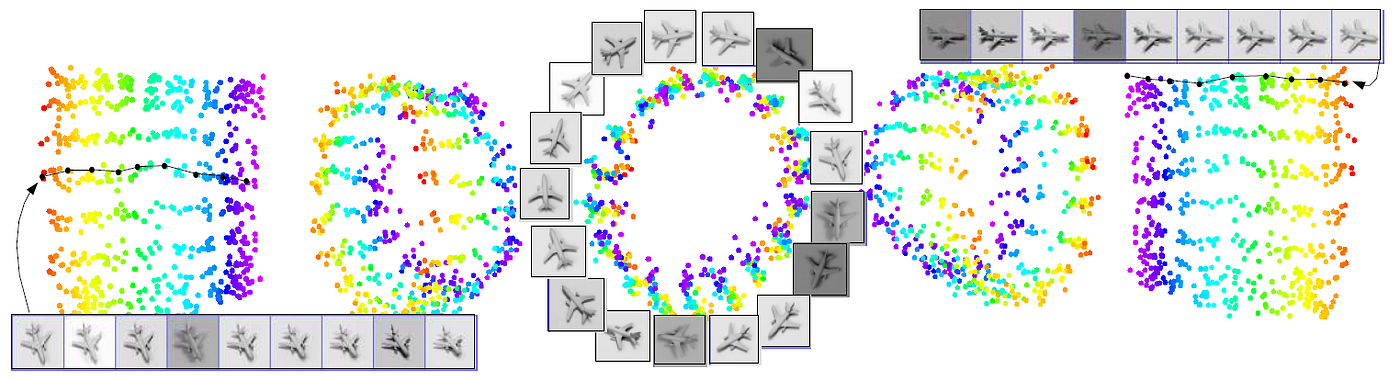# Review — DrLIM: Dimensionality Reduction by Learning an Invariant Mapping

## DrLIM: Contrastive Learning for Dimensionality Reduction

In this story, Dimensionality Reduction by Learning an Invariant Mapping, (DrLIM), by New York University, is reviewed. This is a paper by Prof. LeCun. Originally, this method is proposed for Face Recognition in 2005 CVPR. In this paper:

• A method called Dimensionality Reduction by Learning an Invariant Mapping (DrLIM) is used to map the data evenly to the output manifold.
• The learning relies solely on neighborhood relationships and does not require any distance measure in the input space.

This is a paper in 2006 CVPR with over 2800 citations. (Sik-Ho Tsang @ Medium) This is one basic paper for contrastive learning, and contrastive learning is one key knowledge to know for self-supervised learning.

# Outline

1. The Contrastive Loss Function in General Form
2. The Contrastive Loss Function in Exact Form
3. Spring Model Analogy
4. Network Architecture for GW
5. Experimental Results

# 1. The Contrastive Loss Function in General Form

• Consider the set I of high dimensional training vectors ~Xi, there is a set S ~Xi of training vectors that are deemed similar to ~Xi.
• Let Y be a binary label assigned to this pair.
• Y=0 if ~X1 and ~X2 are deemed similar.
• Y=1 if they are deemed dissimilar.
• Define the parameterized distance function to be learned DW between ~X1, ~X2 as the euclidean distance between the outputs of GW:
• where GW is convolutional neural network (CNN) for MNIST and 2-layer fully connected layer network for airplane images in NORB dataset.
• Then the loss function in its most general form is:
• where (Y, ~X1, ~X2)^i is the i-th labeled sample pair.
• LS is the partial loss function for a pair of similar points.
• LD is the partial loss function for a pair of dissimilar points,
• P is the number of training pairs (which may be as large as the square of the number of samples).

LS and LD must be designed such that minimizing L with respect to W would result in low values of DW for similar pairs and high values of DW for dissimilar pairs.

# 2. The Contrastive Loss Function in Exact FormGraph of the loss function L against the energy DW. The dashed (red) line is the loss function for the similar pairs and the solid (blue) line is for the dissimilar pairs.

## 2.1. Exact Loss Function

• The exact loss function is:
• where m>0 is a margin. The margin defines a radius around GW(~X).

The contrastive term involving dissimilar pairs, LD, is crucial. Simply minimizing DW( ~X1, ~X2) over the set of all similar pairs will usually lead to a collapsed solution.

• Most energy-based models require the use of an explicit contrastive term in the loss function.

## 2.2. Training

• To train, prior knowledge is used to pair the samples as similar samples and dissimilar samples.
• All the pairs are combined to form the labeled training set.
• The above overall loss function is used to update W for each sample pair.

# 3. Spring Model Analogy

• The outputs of GW can be thought of as masses attracting and repelling each other with springs. Consider the equation of a spring:
• where F is the force, K is the spring constant and X is the displacement of the spring from its rest length.

## 3.1. Attracting Force

• A spring is attract-only if its rest length is equal to zero.
• Attractive force is applied to similar pairs (blue & black points).
• The loss function LS(W, ~X1, ~X2) associated with similar pairs:

## 3.2. Repelling Force

• A spring is said to be m-repulse-only if its rest length is equal to m.
• Thus two points that are connected with a m-repulse-only spring will be pushed apart if X is less than m.
• However this spring has a special property that if the spring is stretched by a length X>m, then no attractive force brings it back to rest length.
• The partial loss function LD:
• The force is maximum when DW=0 and absent when DW=m.

## 3.3. EquilibriumThe situation where a point is pulled by other points in different directions, creating equilibrium.

Each point is connected by attract-only springs to similar points, and is connected by m-repulse-only spring to dissimilar points.

# 4. Network Architecture for GW

• First of all, Siamese network is used.

## 4.1. Network Architecture for MNIST

• To be brief, there are 2 convolutions. In between, there is a subsampling layer. At the end, there is a fully connected layer.
• The network is similar to LeNet.

## 4.2. Network Architecture for Airplane Images in NORB Dataset

• 2-layer fully connected layer network is used.
• The number of hidden and output units used was 20 and 3 respectively.

# 5. Experimental Results

## 5.1. MNIST

• The training set is built from 3000 images of the handwritten digit 4 and 3000 images of the handwritten digit 9 chosen randomly from the MNIST dataset.
• Approximately 1000 images of each digit comprised the test set.
• Each sample ~Xi was paired with its 5 nearest neighbors.
• All other possible pairs were labeled dissimilar, producing 30,000 similar pairs and on the order of 18 million dissimilar pairs.
• The mapping of the test set to a 2D manifold is shown above.
• The lighter-colored blue dots are 9’s and the darker-colored red dots are 4’s.

An overall organization that is primarily determined by the slant angle of the samples. The samples are spread rather uniformly in the populated region.

## 5.2.1. DrLIM

• In the distorted set, 3000 images of 4’s and 3000 images of 9’s are horizontally translated by -6, -3, 3, and 6 pixels and combined with the originals, producing a total of 30,000 samples.
• The 2000 samples in the test set were distorted in the same way.

The output points are clustered according to the translated position of the input sample. Within each cluster, however, the samples are well organized and evenly distributed.

## 5.2.2. LLE

• For comparison, the LLE algorithm was used.

There is no global coherence in the embedding.

## 5.2.3. DrLIM Considering Translation

• Each sample paired with (a) its 5 nearest neighbors, (b) its 4 translations, and (c) the 4 translations of each of its 5 nearest neighbors.
• As desired, there is no organization on the basis of translation; in fact, translated versions of a given character are all tightly packed in small regions on the manifold.

Similar characters are mapped to nearby areas, regardless of their shift.

## 5.3. Airplane Images in DORB Dataset

Clearly, the 3D embedding by LLE is NOT invariant to lighting, and the organization of azimuth and elevation does not reflect the real topology neighborhood graph.DrLIM learned a mapping to 3d space for images of a single airplane (The output manifold is shown under five different viewing angles)
• The manifold is roughly cylindrical with a systematic organization: along the circumference varies azimuth of camera in the viewing half-sphere.
• Along the height varies the camera elevation in the viewing sphere.
• The mapping is invariant to the lighting condition, thanks to the prior knowledge built into the neighborhood relationships.

## Reference

[2006 CVPR] [DrLIM]
Dimensionality Reduction by Learning an Invariant Mapping

## Data Visualization

2002 [SNE] 2006 [Autoencoder] [DrLIM] 2007 [UNI-SNE] 2008 [t-SNE]

--

--